Next: Cooperativity Up: PROPERTIES OF REARRANGEMENT PATHWAYS Previous: Introduction   Contents

# Localisation

The outcome of a pathway calculation for an atomic system will generally be a set of intermediate geometries, and the corresponding energies, for points along the two unique steepest-descent paths that link a transition state to two local minima. This discrete representation is a convenient starting point for our analysis of localisation and cooperativity. We number the structures along the path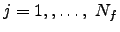starting from one of the two minima and reversing the other steepest-descent path, so that structure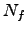corresponds to the other minimum. The transition state then lies somewhere between frames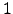and. We define the three-dimensional vector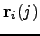to contain the Cartesian coordinates of atom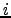for structure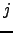, i.e.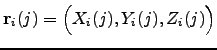, where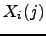is the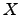coordinate of atomin structure, etc.

For each atomwe also define the displacement between structures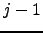andas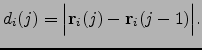(5.1)

Hence the sum of displacements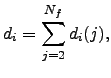(5.2)

is an approximation to the integrated path length for atom, which becomes increasingly accurate for smaller step sizes. The total integrated path length,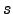, is approximated as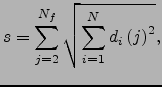(5.3)

where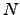is the total number of atoms.is a characteristic property of the complete path, and is expected to correlate with parameters such as the curvature and barrier height for short paths [178,9,177].

The set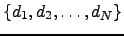containing allvalues of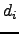will be denoted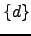, and analogous notation will be used for other sets below. We will also refer to the frequency distribution function, which can provide an alternative representation of such data . For example, the frequency distribution function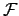for a given continuous variable,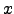, tells us thatoccurs in a certain interval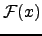times.

Our objective in the present analysis is to provide a more detailed description of the degree of localisation' and cooperativity' corresponding to a given pathway. The first index we consider is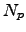, which is designed to provide an estimate of how many atoms participate in the rearrangement. We will refer to a rearrangement as localised if a small fraction of the atoms participate in the rearrangement, and as delocalised in the opposite limit. The second index we define,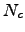,is intended to characterise the number of atoms that move simultaneously, i.e. cooperatively. We will refer to a rearrangement as cooperative if most of the atoms that participate in the rearrangement move simultaneously, and as uncooperative otherwise.

The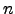th moment about the mean for a data set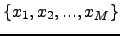is the expectation value of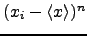, where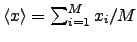and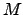is the number of elements in the set. Hence for the setdefined above we define the moments,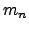, as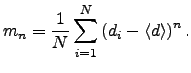(5.4)

The kurtosis of the setis then defined as the dimensionless ratio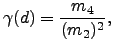(5.5)

and provides a measure of the shape of the frequency distribution function corresponding to. If only one of the atoms moves, or all atoms except one move by the same amount, then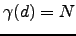. Alternatively, if half the atoms move by the same amount whilst the others are stationary, then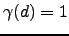. Hence, a distribution with a broad peak and rapidly decaying tails will have a small kurtosis,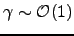, while a distribution with a sharp peak and slowly decaying tails will have a larger value (Figure 3.1). The kurtosis can therefore identify distributions that contain large deviations from the average value . For comparison, a Gaussian distribution has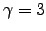and a uniform distribution has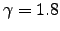.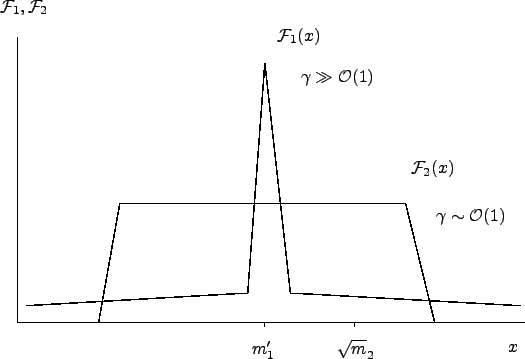The above results show that the kurtosis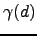can be used to quantify the degree of localisation or delocalisation of a given rearrangement. However, it has the serious disadvantage that highly localised and delocalised mechanisms both have large values of. Since we are interested in estimating the number of atoms that move relative to the number with small or zero displacements, a better approach is to use moments taken about the origin, rather than about the mean, i.e.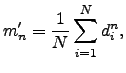(5.6)

following Stillinger and Weber . Note that while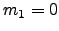, the first moment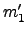is the mean value. We therefore estimate the number of atoms that participate in the rearrangement,, as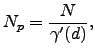(5.7)

where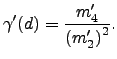(5.8)

For the system withatoms, if only one atom moves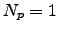, while if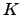atoms move by the same amount,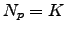.

A similar index tohas been employed in previous work [9,180,19] using only the displacements between the two local minima, which corresponds to taking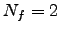in Equation 3.2. Usingvalues based upon a sum of displacements that approximates the integrated path length for atom, rather than the overall displacement between the two minima, better reflects the character of the rearrangement, as it can account for the nonlinearity of the pathway. To describe this property more precisely we introduce a pathway nonlinearity index defined by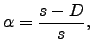(5.9)

where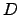is the Euclidean distance  between the endpoints,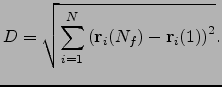(5.10)

We calculated the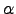values for a database of 31,342 single transition state pathways of LJ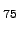(hereafter referred to as the LJdatabase). The average value ofwas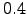with a standard deviation of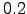, and, hence, there is a significant loss of information if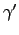is calculated only from the endpoints using. Comparison of the two indices for the LJdatabase revealed many examples where neglect of intermediate structures produces a misleading impression of the number of atoms that move. The definition in Equation 3.7 is therefore suggested as an improvement on previous indices of localisation [9,152,180,19].Next: Cooperativity Up: PROPERTIES OF REARRANGEMENT PATHWAYS Previous: Introduction   Contents
Semen A Trygubenko 2006-04-10